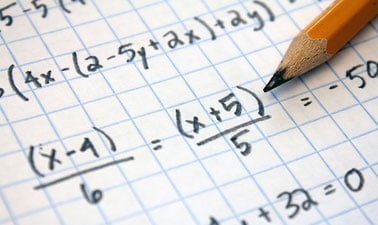# College Algebra

This College Algebra course will cover fundamental concepts of algebra required to interpret a variety of functions and equations.### There is one session available:

After a course session ends, it will be archived.
Starts Oct 22
Estimated 8 weeks
8–12 hours per week
Self-paced
Free

This College Algebra course will cover fundamental concepts of algebra required to interpret a variety of functions and equations. Topics within this course include: linear, quadratic, polynomial, rational, exponential, inverse functions and their graphs, linear inequalities, and linear systems of equations. Students who successfully complete this course will demonstrate increased ability in problem-solving and logical thinking.

### At a glance

• Institution: DoaneX
• Subject: Math
• Level: Intermediate
• Prerequisites:
None
• Language: English
• Video Transcript: English

# What you'll learn

Skip What you'll learn

As a student in this course, students will: ****

1. Compute with and categorize Real numbers
2. Solve and apply equations and inequalities
3. Analyze and use graphs and functions
4. Solve systems of equations and inequalities
5. Compute with and factor polynomials and polynomial functions
6. Compute with rational expressions and solve rational equations
7. Compute with and simplify radicals, roots, and complex numbers
8. Solve and graph quadratic functions